# Propagation of Errors

Propagation of Errors.

(1) Error in sum of the quantities : Suppose   z = x + y

Let

Δx = absolute error in measurement of x

Δy = absolute error in measurement of y

Δz = absolute error in calculation of z

The maximum absolute error in z is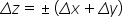Percentage error in the value of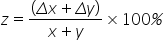(2) Error in difference of the quantities : Suppose z = xy

Let

Δx = absolute error in measurement of x,

Δy = absolute error in measurement of y

Δz = absolute error in calculation of z.

The maximum absolute error in z isPercentage error in the value of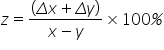(3) Error in product of quantities : Suppose z = x ´ y

Let

Δx = absolute error in measurement of x,

Δy = absolute error in measurement of y

Δz = absolute error in calculation of z.

The maximum fractional error in z is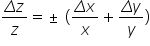Percentage error in the value of z = (Percentage error in value of x) + (Percentage error in value of y)

(4) Error in division of quantities : Suppose z=x / y

Let

Δz = absolute error in measurement of z,

Δx = absolute error in measurement of x

Δy = absolute error in calculation of y.

The maximum fractional error in z is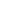Percentage error in the value of z = (Percentage error in value of x) + (Percentage error in value of y)

(5) Error in quantity raised to some power : Suppose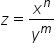Let

Δx = absolute error in measurement of x,

Δy = absolute error in measurement of y

Δz = absolute error in calculation of z

The maximum fractional error in z is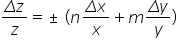Percentage error in the value of z = n (Percentage error in value of x) + m (Percentage error in value of y)

Related Keywords